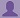SHARE
HELP

To start with, Sr2Jr’s first step is to reduce the expenses related to education. To achieve this goal Sr2Jr organized the textbook’s question and answers. Sr2Jr is community based and need your support to fill the question and answers. The question and answers posted will be available free of cost to all.# Absolute C++

Authors:
Walter Savitch ,kenrick Mock
Exercise:
Programming Projects
Chapter:
C++ Basics
Edition:
5
ISBN:
9780132846813
Question:
4
 Previous Next

###Question

Negotiating a consumer loan is not always straightforward. One form of loan is the discount installment loan, which works as follows. Suppose a loan has a face value of \$1,000, the interest rate is 15%, and the duration is 18 months. The interest is computed by multiplying the face value of \$1,000 by 0.15, yielding \$150. That figure is then multiplied by the loan period of 1.5 years to yield \$225 as the total interest owed. That amount is immediately deducted from the face value, leaving the consumer with only \$775. Repayment is made in equal monthly installments based on the face value. So the monthly loan payment will be \$1,000 divided by 18, which is \$55.56. This method of calculation may not be too bad if the consumer needs \$775 dollars, but the calculation is a bit more complicated if the consumer needs \$1,000. Write a program that will take three inputs: the amount the consumer needs to receive, the interest rate, and the duration of the loan in months. The program should then calculate the face value required in order for the consumer to receive the amount needed. It should also calculate the monthly payment.

###Answer

``````#include <iostream>
using namespace std;

int main()
{
char choice;
do
{
cout.setf(ios::fixed);
cout.setf(ios::showpoint);
cout.precision(2);

double faceValue(0);
double intrestRate(0);
double loanDuration(0);
double yearDuration,intrest,repayment,loan;
cout << "Enter Loan Amount: \$";
cin >> faceValue;
cout << "Enter Interest Rate: ";

cout.setf(ios::fixed);
cout.setf(ios::showpoint);
cout.precision(3);

cin >> intrestRate;

cout << "Enter duration: ";
cin >> loanDuration;

cout.setf(ios::fixed);
cout.setf(ios::showpoint);
cout.precision(2);

yearDuration = (loanDuration/12);
intrest =((faceValue * intrestRate) * yearDuration);
repayment = (faceValue/loanDuration);
loan = (faceValue - intrest);

cout << "Total loan interest: \$" << intrest << ".\n"
<< "Given amount is: \$" << loan << ".\n"
<< "Monthly payment for " << loanDuration
<< " months is: \$" << repayment << ".\n"
<< "If you would like to repeat this procedure,then enter y:\n";
cin >> choice;

} while (choice == 'y' || choice == 'Y');
return 0;
}``````

Output Result:

``````Enter Loan Amount: \$1000
Enter Interest Rate: 15
Enter duration: 150
Total loan interest: \$187500.00.
Given amount is: \$-186500.00.
Monthly payment for 150.00 months is: \$6.67.
If you would like to repeat this procedure,then enter y: n
``````

### Discussions

Post the discussion to improve the above solution.